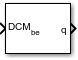# Direction Cosine Matrix to Quaternions

Convert direction cosine matrix to quaternion vector

• Library:
• Aerospace Blockset / Utilities / Axes Transformations

•## Description

The Direction Cosine Matrix to Quaternions block transforms a 3-by-3 direction cosine matrix (DCM) into a four-element unit quaternion vector (q0, q1, q2, q3). Aerospace Blockset™ uses quaternions that are defined using the scalar-first convention. The DCM performs the coordinate transformation of a vector in inertial axes to a vector in body axes. For more information on the direction cosine matrix, see Algorithms.

## Ports

### Input

expand all

Direction cosine matrix to transform the direction cosine matrix to quaternions, specified as a 3-by-3.

Data Types: `double`

### Output

expand all

Quaternion returned by transformation as a 4-by-1 vector.

Data Types: `double`

## Parameters

expand all

Block behavior when the direction cosine matrix is invalid (not orthogonal).

• `Warning` — Displays warning indicating that the direction cosine matrix is invalid.

• `Error` — Displays error indicating that the direction cosine matrix is invalid.

• `None` — Does not display warning or error (default).

#### Programmatic Use

 Block Parameter: `action` Type: character vector Values: `'None'` | `'Warning'` | `'Error'` Default: `'None'`

Data Types: `char` | `string`

Tolerance of the direction cosine matrix validity, specified as a scalar. The block considers the direction cosine matrix valid if these conditions are true:

• The transpose of the direction cosine matrix times itself equals `1` within the specified tolerance (`transpose(n)*n == 1±tolerance`).

• The determinant of the direction cosine matrix equals `1` within the specified tolerance (`det(n) == 1±tolerance`).

#### Programmatic Use

 Block Parameter: `tolerance` Type: character vector Values: `'eps(2)'` | scalar Default: `'eps(2)'`

Data Types: `double`

## Algorithms

The DCM is defined as a function of a unit quaternion vector by the following:

`$DCM=\left[\begin{array}{ccc}\left({q}_{0}^{2}+{q}_{1}^{2}-{q}_{2}^{2}-{q}_{3}^{2}\right)& 2\left({q}_{1}{q}_{2}+{q}_{0}{q}_{3}\right)& 2\left({q}_{1}{q}_{3}-{q}_{0}{q}_{2}\right)\\ 2\left({q}_{1}{q}_{2}-{q}_{0}{q}_{3}\right)& \left({q}_{0}^{2}-{q}_{1}^{2}+{q}_{2}^{2}-{q}_{3}^{2}\right)& 2\left({q}_{2}{q}_{3}+{q}_{0}{q}_{1}\right)\\ 2\left({q}_{1}{q}_{3}+{q}_{0}{q}_{2}\right)& 2\left({q}_{2}{q}_{3}-{q}_{0}{q}_{1}\right)& \left({q}_{0}^{2}-{q}_{1}^{2}-{q}_{2}^{2}+{q}_{3}^{2}\right)\end{array}\right]$`

Using this representation of the DCM, a number of calculations arrive at the correct quaternion. The first of these is to calculate the trace of the DCM to determine which algorithms are used. If the trace is greater than zero, the quaternion can be automatically calculated. When the trace is less than or equal to zero, the major diagonal element of the DCM with the greatest value must be identified to determine the final algorithm used to calculate the quaternion. Once the major diagonal element is identified, the quaternion is calculated.

## Extended Capabilities

### C/C++ Code GenerationGenerate C and C++ code using Simulink® Coder™.

Introduced before R2006a

## Support평가판 신청